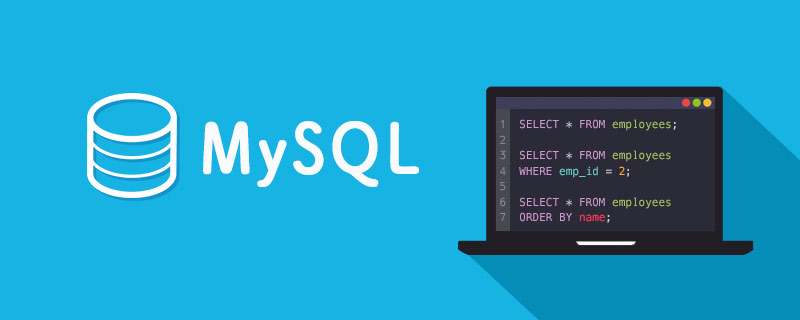# 通过ProxySQL方法提升PHP/MySQL的性能_编程技术_编程开发技术教程

```datadir="/var/lib/proxysql"
{
mysql_ifaces="0.0.0.0:6032"
}
mysql_variables=
{
max_connections=2048
default_query_delay=0
default_query_timeout=36000000
have_compress=true
poll_timeout=2000
interfaces="/var/run/proxysql.sock"
default_schema="information_schema"
stacksize=1048576
server_version="5.5.30"
connect_timeout_server=3000
monitor_history=600000
monitor_connect_interval=60000
monitor_ping_interval=10000
ping_interval_server_msec=120000
ping_timeout_server=500
commands_stats=true
sessions_sort=true
connect_retries_on_failure=10
}
mysql_servers =
(
{
port=3306
}
)
mysql_users:
(
{
}
)```

```<?php
\$host = '...';
\$user = '...';
\$database = '...';
\$charset = 'utf8mb4';
\$socket = '/var/run/proxysql.sock';
\$dsn = "mysql:dbname={\$database};charset={\$charset}";
if (empty(\$_GET['proxysql'])) {
\$dsn .= ";host={\$host}";
} else {
\$dsn .= ';unix_socket={\$socket}';
}
\$dbh = new PDO(\$dsn, \$user, \$password);
\$sql = 'SELECT * FROM foo LIMIT 10';
\$value = \$dbh->query(\$sql);
foreach (\$value as \$v) {
var_dump(\$v);
}
?>```

```shell> ab -k -n 10000 -c 100 "http://path/test.php?proxysql=0"
shell> ab -k -n 10000 -c 100 "http://path/test.php?proxysql=1"```